Want to share your content on R-bloggers? click here if you have a blog, or here if you don't.

### Introduction

This is the 18th post in the series Elegant Data Visualization with ggplot2. In the previous post, we learnt how to modify the legend of plot when `alpha` is mapped to a categorical variable. In this post, we will learn to modify legend

• title
• label
• and bar

So far, we have learnt to modify the components of a legend using `scale_*` family of functions. Now, we will use the `guide` argument and supply it values using the `guide_legend()` function.

### Libraries, Code & Data

We will use the following libraries in this post:

All the data sets used in this post can be found here and code can be downloaded from here.

### Title

#### Title Alignment

The horizontal alignment of the title can be managed using the `title.hjust` argument. It can take any value between `0` and `1`.

• 0 (left)
• 1 (right)

In the below example, we align the title to the center by assigning the value `0.5`.

```ggplot(mtcars) + geom_point(aes(disp, mpg, color = factor(cyl))) +
scale_color_manual(values = c("red", "blue", "green"),
guide = guide_legend(title = "Cylinders", title.hjust = 0.5))```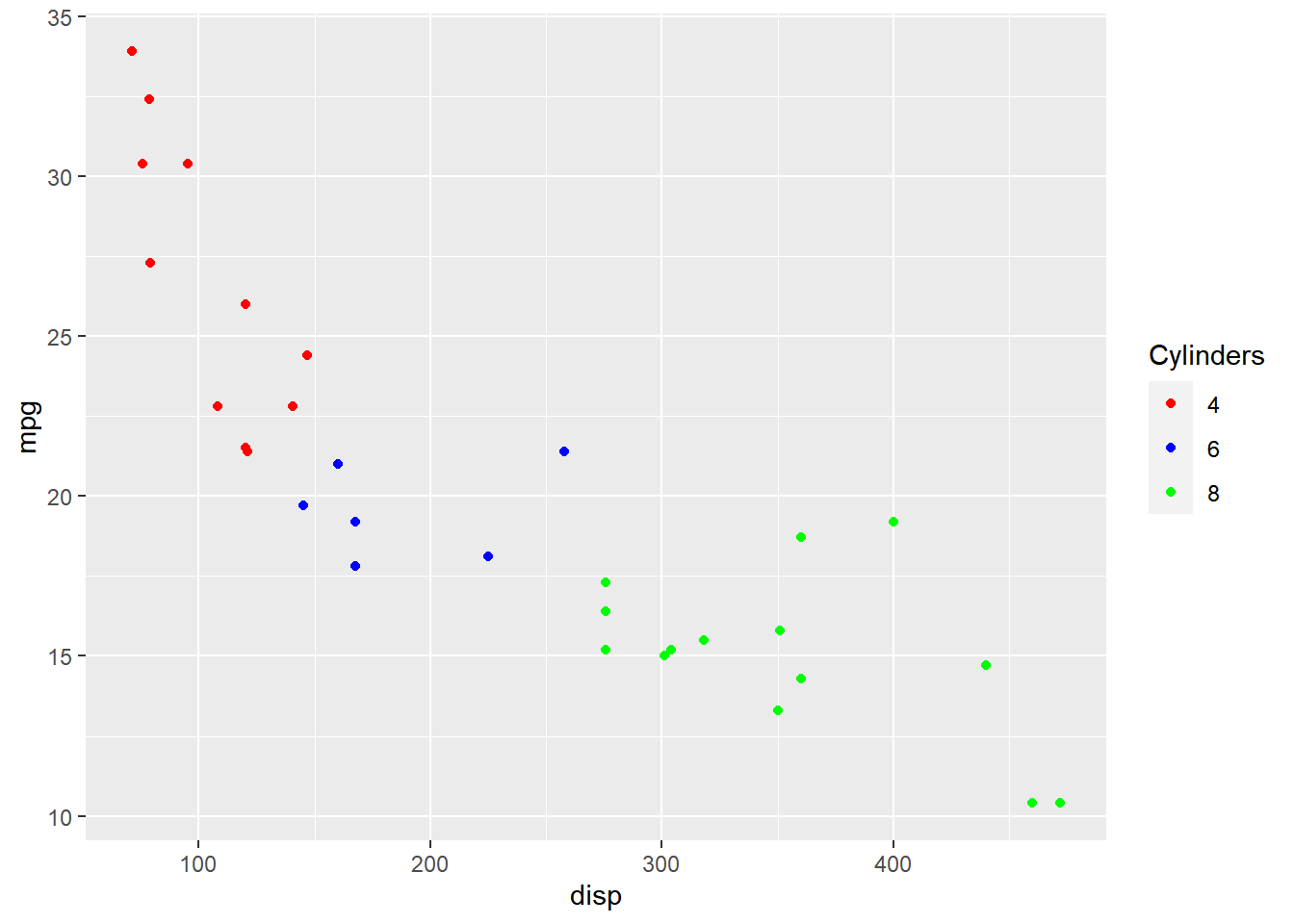#### Title Alignment (Vertical)

To manage the vertical alignment of the title, use `title.vjust`.

```ggplot(mtcars) + geom_point(aes(disp, mpg, color = hp)) +
scale_color_continuous(guide = guide_colorbar(
title = "Horsepower", title.position = "top", title.vjust = 1))```#### Title Position

The position of the title can be managed using `title.posiiton` argument. It can be positioned at:

• top
• bottom
• left
• right
```ggplot(mtcars) + geom_point(aes(disp, mpg, color = factor(cyl))) +
scale_color_manual(values = c("red", "blue", "green"),
guide = guide_legend(title = "Cylinders", title.hjust = 0.5,
title.position = "top"))```## Label

### Label Position

The position of the label can be managed using the `label.position` argument. It can be positioned at:

• top
• bottom
• left
• right

In the below example, we position the label at right.

```ggplot(mtcars) + geom_point(aes(disp, mpg, color = factor(cyl))) +
scale_color_manual(values = c("red", "blue", "green"),
guide = guide_legend(label.position = "right"))```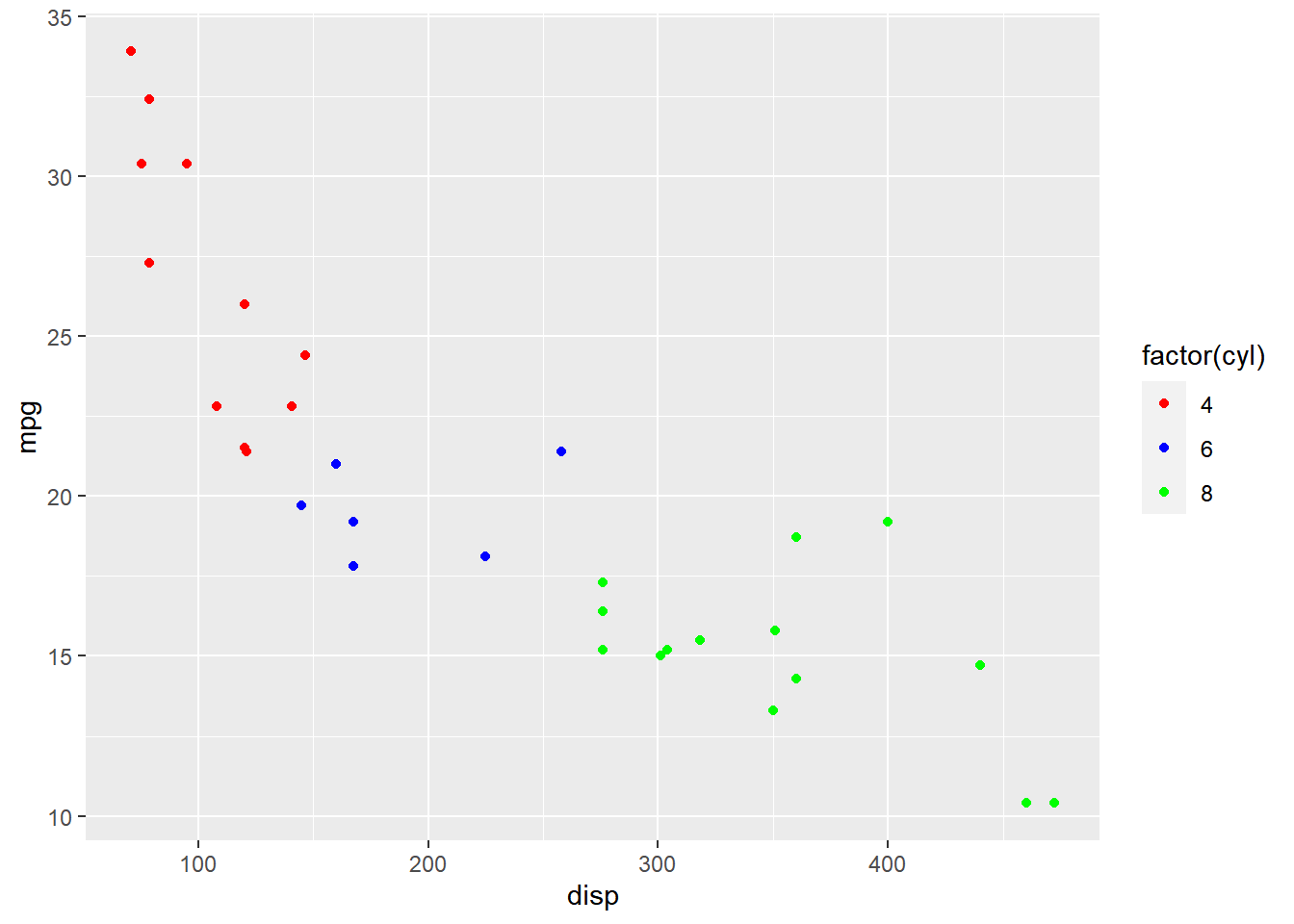### Label Alignment

The horizontal alignment of the label can be managed using the `label.hjust` argument. It can take any value between `0` and `1`.

• 0 (left)
• 1 (right)

In the below example, we align the label to the center by assigning the value `0.5`.

• alignment
• 0 (left)
• 1 (right)
```ggplot(mtcars) + geom_point(aes(disp, mpg, color = factor(cyl))) +
scale_color_manual(values = c("red", "blue", "green"),
guide = guide_legend(label.hjust = 0.5))```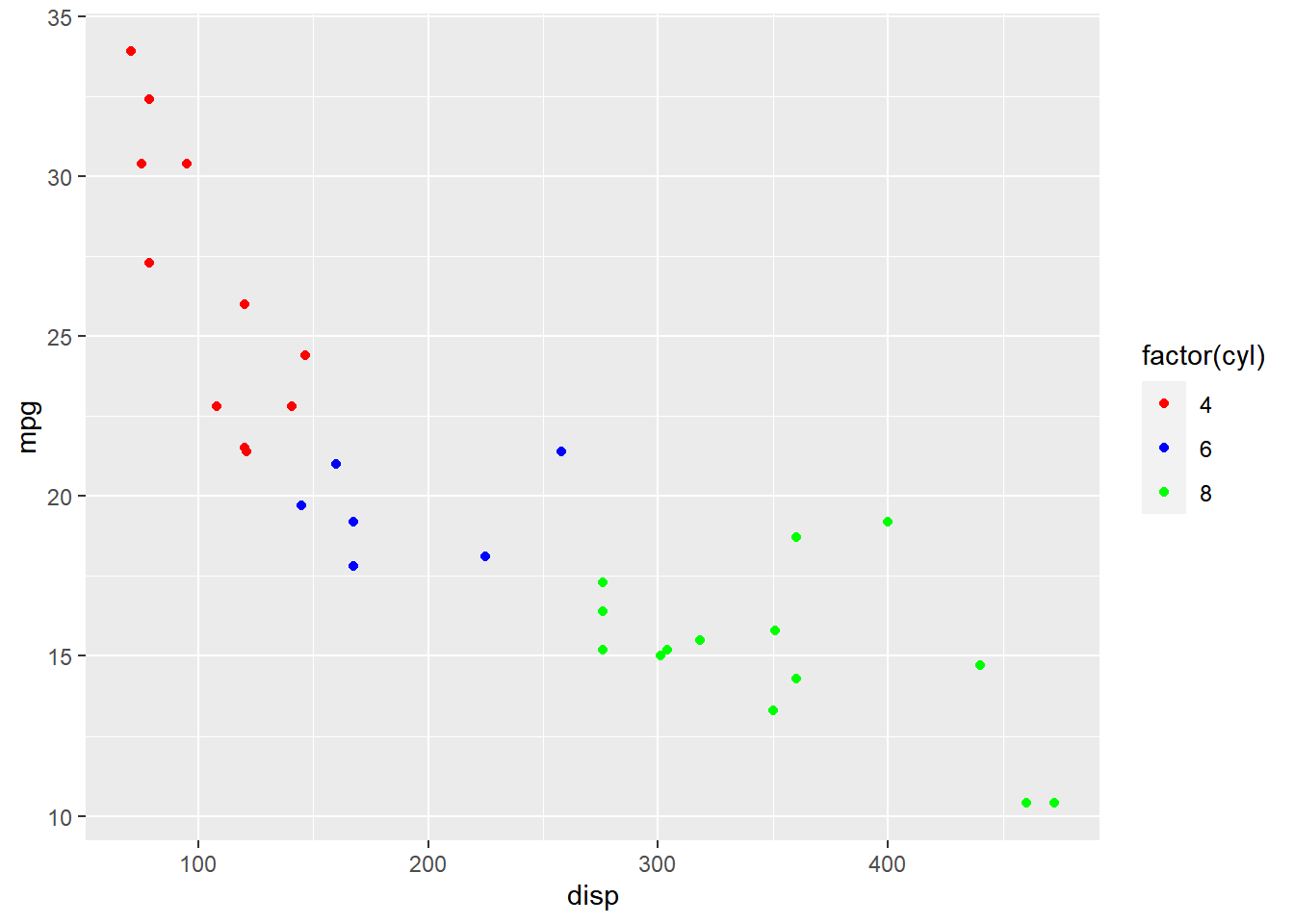#### Labels Alignment (Vertical)

The vertical alignment of the label can be managed using the `label.vjust` argument.

```ggplot(mtcars) +
geom_point(aes(disp, mpg, color = hp)) +
scale_color_continuous(guide = guide_colorbar(
label.vjust = 0.8))```### Direction

The direction of the label can be either horizontal or veritcal and it can be set using the `direction` argument.

```ggplot(mtcars) + geom_point(aes(disp, mpg, color = factor(cyl))) +
scale_color_manual(values = c("red", "blue", "green"),
guide = guide_legend(direction = "horizontal"))```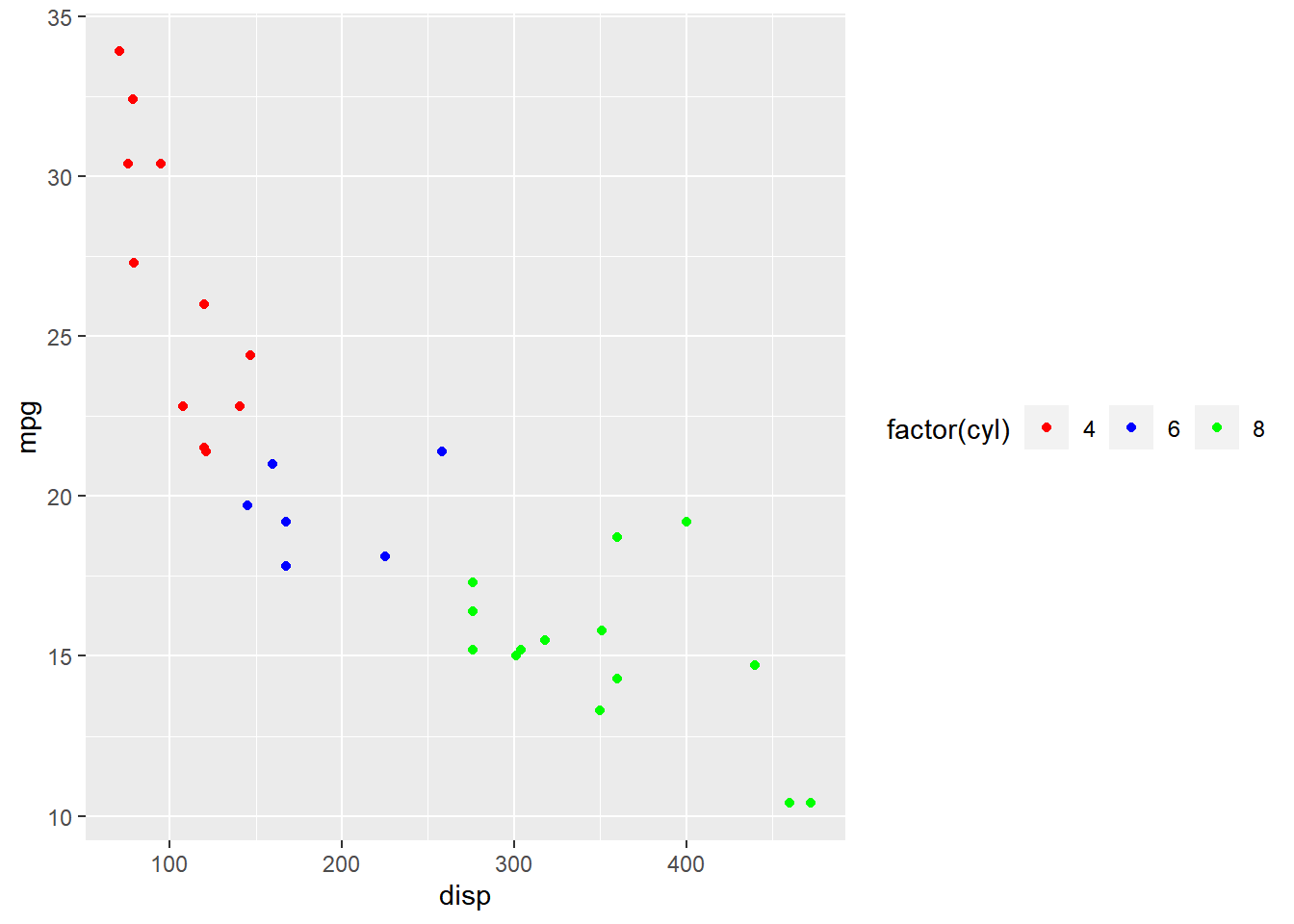### Rows

The label can be spread across multiple rows using the `nrow` argument. In the below example, the label is spread across 2 rows.

```ggplot(mtcars) + geom_point(aes(disp, mpg, color = factor(cyl))) +
scale_color_manual(values = c("red", "blue", "green"),
guide = guide_legend(nrow = 2))```### Reverse

The order of the labels can be reversed using the `reverse` argument. We need to supply logical values i.e. either `TRUE` or `FALSE`. If `TRUE`, the order will be reversed.

```ggplot(mtcars) + geom_point(aes(disp, mpg, color = factor(cyl))) +
scale_color_manual(values = c("red", "blue", "green"),
guide = guide_legend(reverse = TRUE))```### Putting it all together…

```ggplot(mtcars) + geom_point(aes(disp, mpg, color = factor(cyl))) +
scale_color_manual(values = c("red", "blue", "green"),
guide = guide_legend(title = "Cylinders", title.hjust = 0.5,
title.position = "top", label.position = "right",
direction = "horizontal", label.hjust = 0.5, nrow = 2, reverse = TRUE)
)```### Legend Bar

So far we have looked at modifying components of the legend when it acts as a guide for `color`, `fill` or `shape` i.e. when the aesthetics have been mapped to a categorical variable. In this section, you will learn about `guide_colorbar()` which will allow us to modify the legend when the aesthetics are mapped to a continuous variable.

### Plot

Let us start with a scatter plot examining the relationship between displacement and miles per gallon from the mtcars data set. We will map the color of the points to the `hp` variable.

```ggplot(mtcars) +
geom_point(aes(disp, mpg, color = hp))```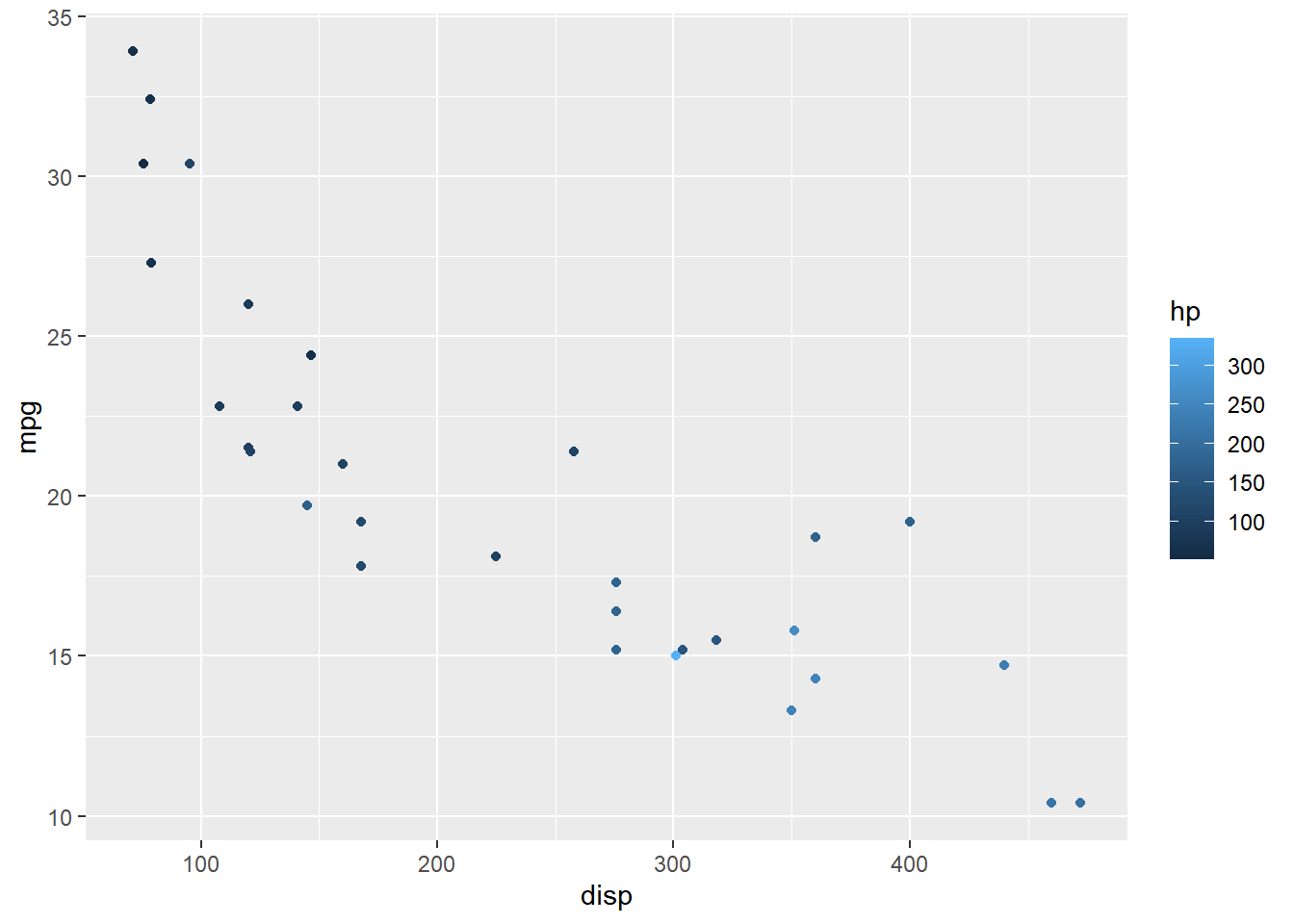### Width

The width of the bar can be modified using the `barwidth` argument. It is used inside the `guide_colorbar()` function which itself is supplied to the `guide` argument of `scale_color_continuous()`.

```ggplot(mtcars) +
geom_point(aes(disp, mpg, color = hp)) +
scale_color_continuous(guide = guide_colorbar(
barwidth = 10))```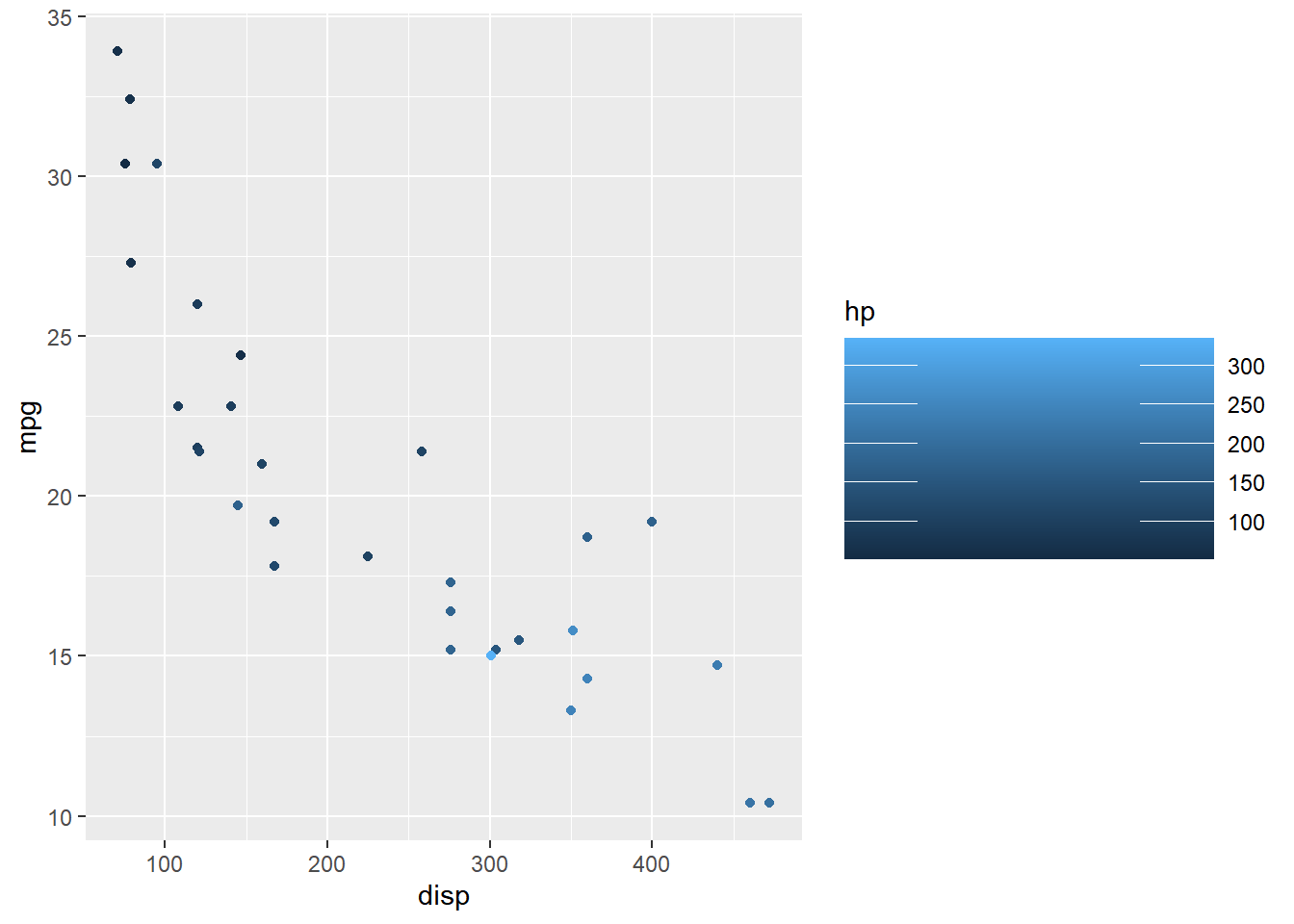### Height

Similarly, the height of the bar can be modified using the `barheight` argument.

```ggplot(mtcars) +
geom_point(aes(disp, mpg, color = hp)) +
scale_color_continuous(guide = guide_colorbar(
barheight = 3))```### Bins

The `nbin` argument allows us to specify the number of bins in the bar.

```ggplot(mtcars) +
geom_point(aes(disp, mpg, color = hp)) +
scale_color_continuous(guide = guide_colorbar(
nbin = 4))```### Ticks

The ticks of the bar can be removed using the `ticks` argument and setting it to `FALSE`.

```ggplot(mtcars) +
geom_point(aes(disp, mpg, color = hp)) +
scale_color_continuous(guide = guide_colorbar(
ticks = FALSE))```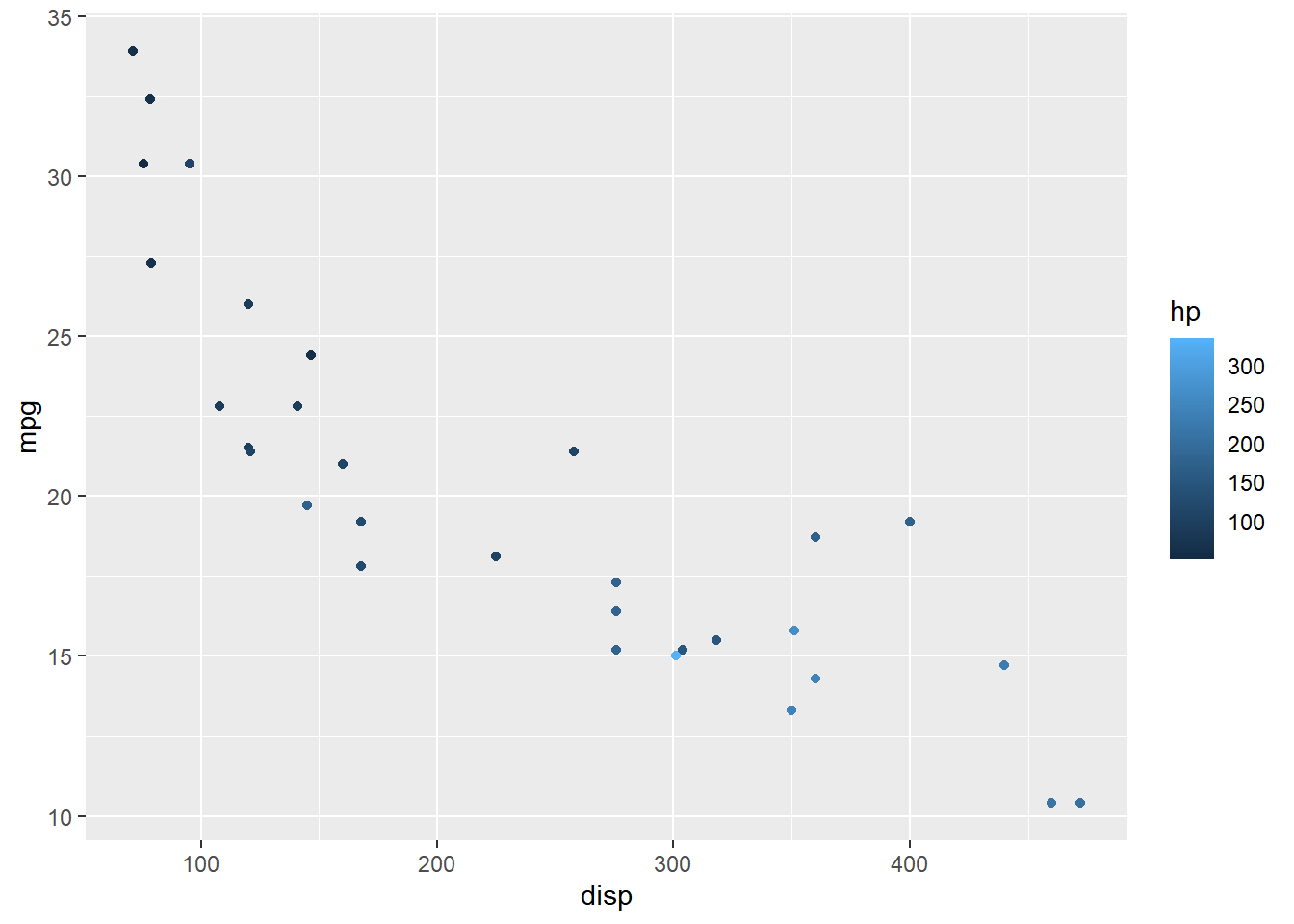### Upper/Lower Limits

The upper and lower limits of the bars can be drawn or undrawn using the `draw.ulim` and `draw.llim` arguments. They both accept logical values.

```ggplot(mtcars) +
geom_point(aes(disp, mpg, color = hp)) +
scale_color_continuous(guide = guide_colorbar(
draw.ulim = TRUE, draw.llim = FALSE))```### Guides: Color, Shape & Size

The `guides()` function can be used to create multiple legends to act as a guide for `color`, `shape`, `size` etc. as shown below. First, we map color, shape and size to different variables. Next, in the `guides()` function, we supply values to each of the above aesthetics to indicate the type of legend.

```ggplot(mtcars) +
geom_point(aes(disp, mpg, color = hp,
size = qsec, shape = factor(gear))) +
guides(color = "colorbar", shape = "legend", size = "legend")```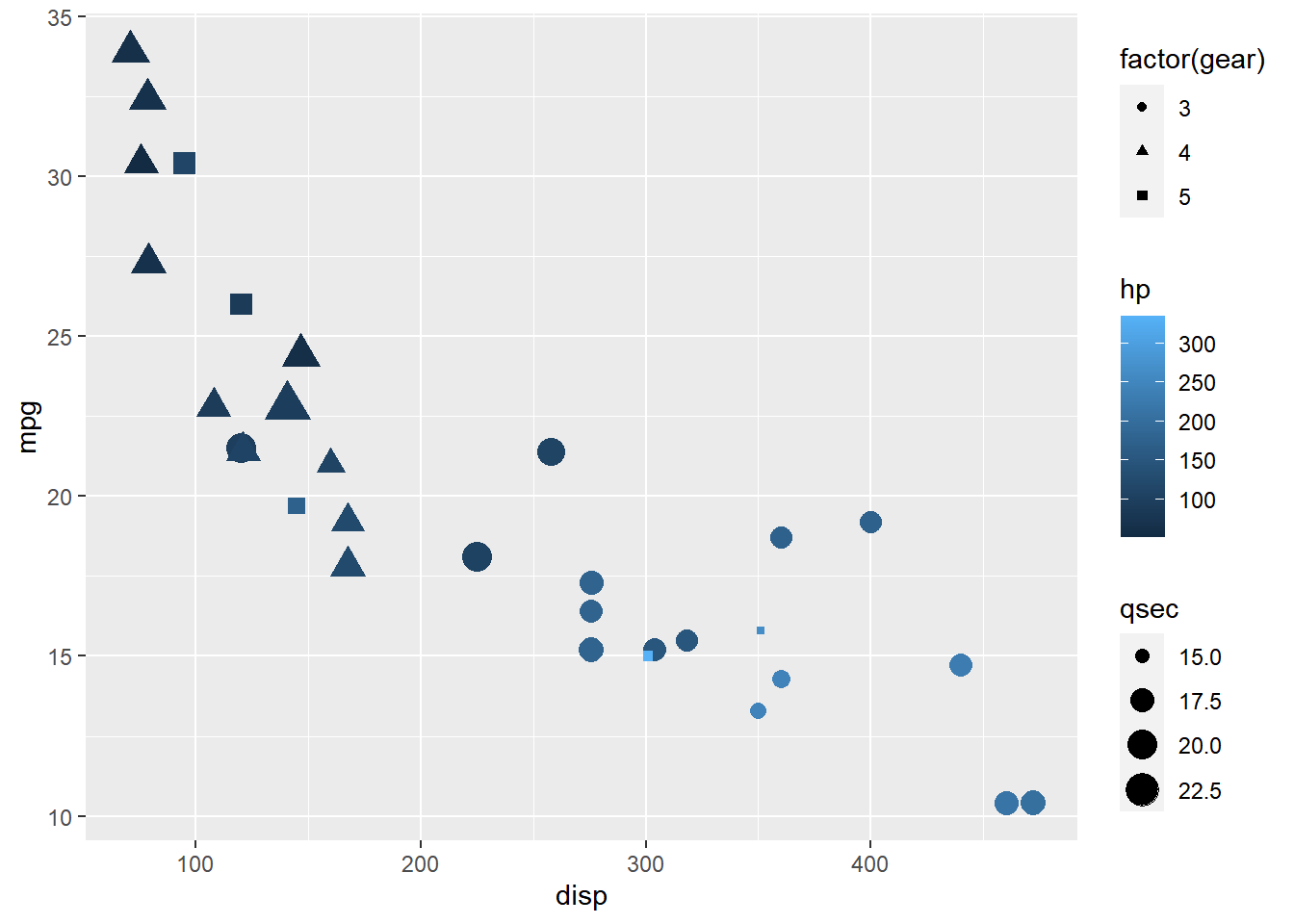### Guides: Title

To modify the components of the different legends, we must use the `guide_*` family of functions. In the below example, we use `guide_colorbar()` for the legend acting as guide for color mapped to a continuous variable and `guide_legend()` for the legends acting as guide for shape/size mapped to categorical variables.

```ggplot(mtcars) +
geom_point(aes(disp, mpg, color = hp, size = wt, shape = factor(gear))) +
guides(color = guide_colorbar(title = "Horsepower"),
shape = guide_legend(title = "Weight"), size = guide_legend(title = "Gear")
)```### Summary

In this post, we will learn to modify legend

• title
• label
• and bar

### Up Next..

In the next post, we will learn faceting.